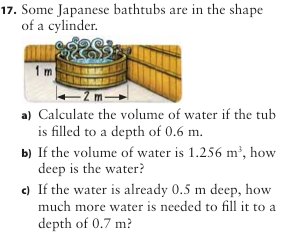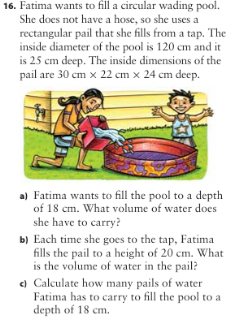## Tuesday, March 15, 2011

### Hanna's Volume Scribe Post

18. Suki has 30 small linking cubes.

a) She wants to use 18 of them to make a large cube. Is this possible? Why or why not?

b) What number of linking cubes would she use to construct the largest cube she can
possibly make?

a) It is not possble to make a large cube with only 18 linking cube because there isn't a whole number that can make 18 when cubed. To get 18, each side of the cube should have 2.6207413942088964 cubes.

b) v = s x s x s
v =1y x 1y x 1y
v = 1y³

v = s x s x s
v = 2 x 2 x 2
v =

v = s x s x s
v = 3 x 3 x 3
v = 27³

v = s x s x s
v = 4 x 4 x 4
v = 64³

The largest cube Suki can make with only 30 linking cubes uses 27 cubes.

19. Melissa has three glass vases. She wants to use one as a decorative fish tank for Harvey the
guppy. Which will give Harvey the most water to swim in?Cube Tank

v = s x s x s
v = 7cm x 7cm x 7cm
v= 343cm³

Rectangular Prism Tank

v = l x w x h
v = 10cm x 9cm x 4cm
v = 360cm³

Triangular Prism Tank

v = b x h(1) / 2 x h(2)
v = 7cm x 5cm / 2 x 21cm
v = 367.5cm³

The triangular prism tank can contain the most water.

Cylinder Volume and Volume Problemsa) r = d ÷ 2
r = 2m ÷ 2
r = 1m

v = π x r² x h
v = 3.14 x 1² x 0.6

v = 1.884m³

b) h = v ÷ a of b

h = 1.256 ÷ 3.14

h = 0.4m

c) v = pi x r² x h

v = 3.14 x 1² x 0.2

v = 0.628m³d ÷ 2

r = 120 ÷ 2
r = 60cm

a) v = pi x r² x h

v = 3.14 x 60² x 18

v = 3391.2cm³

b) v = l x w x h

v = 30 x 22 x 24

v = 15840cm³

c) 15840cm³ x 1 = 15840cm³

15840cm³ x 2 = 31680cm³

15840cm³ x 3 = 47520cm³

She would have to fill it 3 times

1.2.